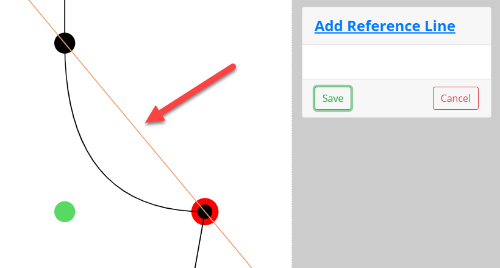Reference lines are different from regular lines. They don't have start and end points. They will originate from a single point and then draw out from there.

Before we go through the process, familiarize yourself with these choices.

## Line Width

### Ray

A Ray will originate on the selected point and draw out in one direction from there.

### Infinite Line

An Infinite Line will originate on the selected point and draw out in both directions from there.

## Line Direction

You have three different directions to choose from.

### Horizontal

The reference line will be drawn horizontally through the selected point.

### Vertical

The reference line will be drawn vertically through the selected point.

### On Vector

The reference line will be drawn through the reference point with a second point used as the vector.

## Process

### Ray Horizontal

Select a starting point. This can be a key point, reference point or curve point.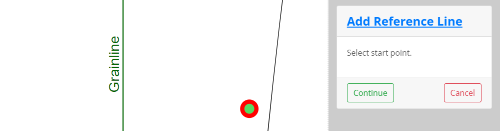In this example, we are choosing a Ray.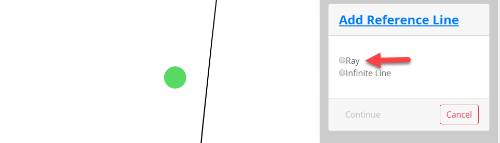Next we will choose Horizontal.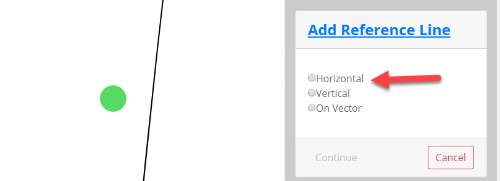The program will draw a line extending from the point horizontally in one direction. If you want it to go the other direction, select Other Side.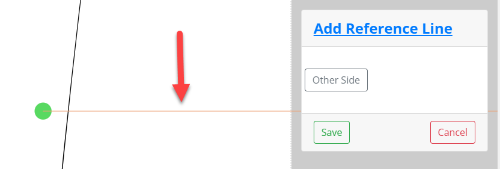## Infinite Line Vertical

In this example, we'll select and Infinite Line vertically.

Select a starting point. This can be a key point, reference point or curve point.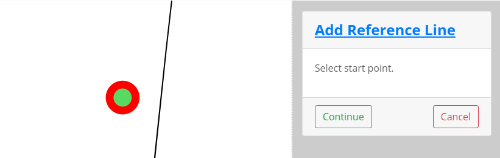We will choose to draw an Infinite Line.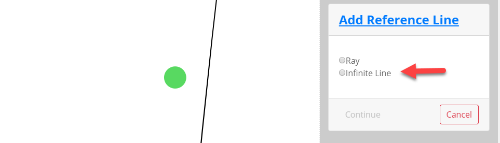Next we will choose Vertical.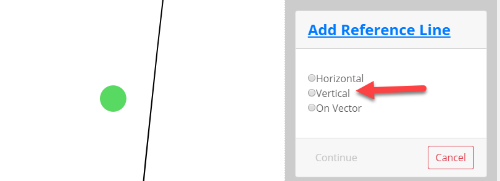The program will draw a line originating at the point extending in both directions vertically.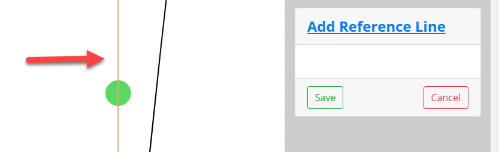## On Vector

Lastly, we'll draw an Infinite Line that goes through a point using another point as the vector.

Select a starting point. This can be a key point, reference point or curve point.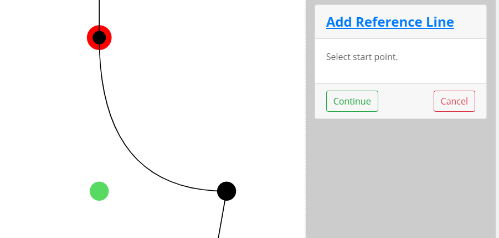We will choose an Infinite Line.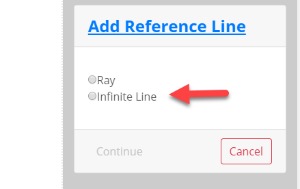Next we will choose On Vector.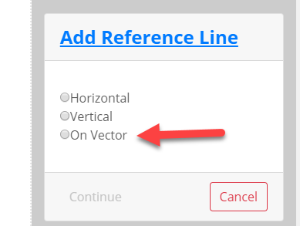The system will ask for us to select a vector point.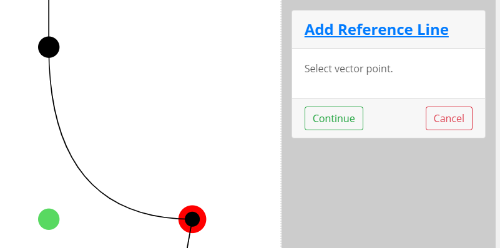The program will draw a line originating at the point extending in both directions using the second point for the vector.